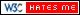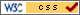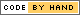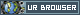# Parallel Processing

## OpenMP

Once the code was implemented and tested thoroughly, next task was to implement multi-threading using OpenMP. A number of OpenMP directives were explored from the class learnings to achieve higher performance. It was observed that a total of 11 seconds were taken to execute the program. On analyzing the system performance, it was noticed that only one core was utilized while the rest of the three cores were idle. Thus to improve the efficiency, OpenMP was used such that all four cores are utilized fully. This resulted in a reduction of latency by 45%. In OpenMP, multiple threads are launched for a single application. The threads are allocated to different processors at run time. We follow four step procedure while using OpenMP:

1.Setup up a team of parallel threads

2.Decide what to run in parallel

3.Check the results

4.Check the speed-up

A simple illustration of how the fork join model works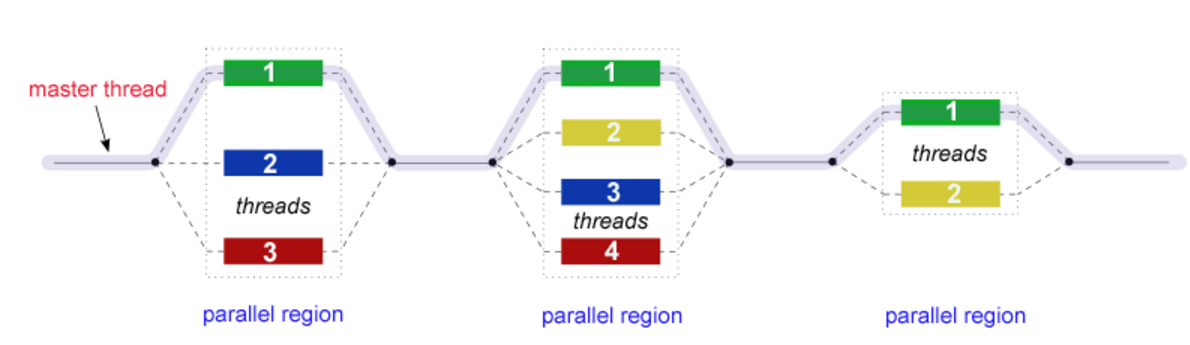Multiprocessing in action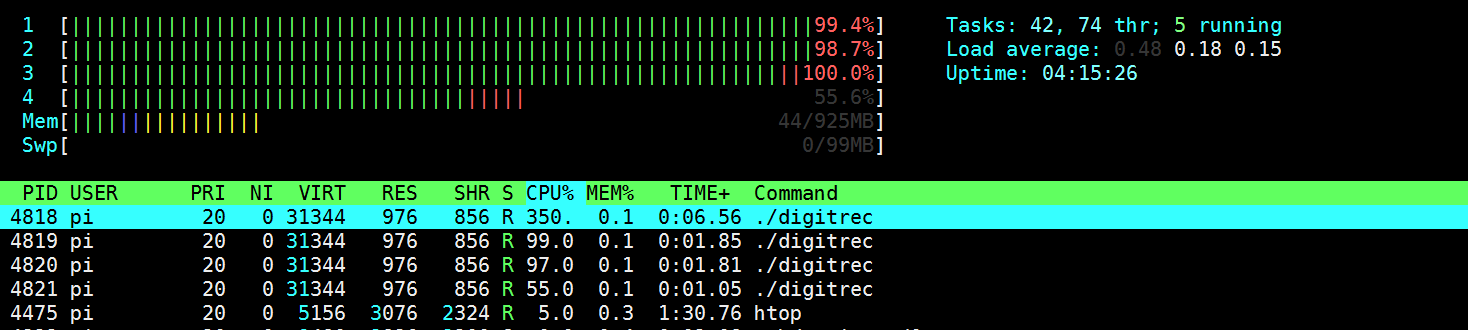Figure 2: Htop results

## Testing

An incremental design testing was adopted as the primary testing strategy such that each sub-section that was implemented was first thoroughly tested for its effectiveness. At first, each digit was tested one after the other to check the effectiveness of the code. Once tested, later the test input size was made large.

In K-nearest neighbor (K-nn) algorithm for digit recognition, k = 4 was chosen. This is because it gave the highest accuracy of 95%. With k=2, an accuracy of 91% was achieved while with k = 3, accuracy of 94% was obtained. Thus, it was observed that the accuracy was comparably good for k< 8, however beyond this value accuracy drops below 90%.

In case of OpenMP, a number of different optimizations were tested using various OpenMP directives such as making the result of the x-or operation shared since all threads need to perform this operation. In executing this, however it was observed that even though CPU utilization was more i.e. 2.215 which is more than 1.7 in the earlier case, the time taken for execution is now 10secs which is almost equivalent to the code without OpenMP. Another optimization that was tested was using reduction operator for x-or between test and train data. Since multiple train data is x-or with a single test data, the test was made private for each thread. A better performance was obtained with an execution time of 3secs but the accuracy was now below 50 %. After analyzing the program, it was found the accuracy dropped because the reduction operator ignores any value and starts from zero.

## Code Appendix

### Optimized version of Knn Algorithm 

 ``` 1 2 3 4 5 6 7 8 9 10 11 12 13 14 15 16 17 18 19 20 21 22 23 24 25 26 27 28 29 30 31 32 33 34 35 36 37 38 39 40 41 42 43 44 45 46 47 48 49 50 51 52 53 54 55 56 57 58 59 60 61 62 63 64 65 66 67 68 69 70 71 72 73 74 75 76 77 78 79 80 81 82 83 84 85 86 87 88 89 90 91 92 93 94 95 96 97 98 99 100 101 102 103 104 105 106 107 108 109 110 111 112 113 114 115 116 117 118 119 120 121 122 123 124 125 126 127 128 129``` ```//========================================================================== //Digit Recognition using OpenMP //========================================================================== // @brief: A k-nearest-neighbors implementation for digit recognition // Name: Siddharth Mody, Mayank Sharma Net-id: sbm99, ms3272 // Class: ECE5990 #include "training_data.h" #include "digitrec.h" #include #include #include using namespace std; int digitrec( long long int input ) { int omp_get_max_threads(void); // This array stores K minimum distances per training set int knn_set[K_CONST]; int max_threads; max_threads = omp_get_max_threads(); // cout << "max_threads" << max_threads; //===================================================================================== // Initialize the knn set //===================================================================================== // setup open_mp thread team OMP # pragma omp parallel num_threads(max_threads) for ( int i = 0; i < 10; ++i ) # pragma omp for for ( int k = 0; k < K_CONST; ++k ) // Note that the max distance is 49 knn_set[i][k] = 50; int i,j; long long int training_instance; // # pragma omp parallel for private (j) shared (training_instance) for ( int j = 0; j < 10; j++ ) { # pragma omp parallel for private (i) shared (training_instance) for ( int i = 0; i < TRAINING_SIZE; ++i ) { // Read a new instance from the training set long long int training_instance = training_data[j * TRAINING_SIZE + i]; // Update the KNN set update_knn( input, training_instance, knn_set[j] ); } } // Compute the final output return knn_vote( knn_set ); } //----------------------------------------------------------------------- // update_knn function //----------------------------------------------------------------------- // Given the test instance and a (new) training instance, this // function maintains/updates an array of K minimum // distances per training set. void update_knn( long long int test_inst, long long int train_inst, int min_distances[K_CONST] ) { long long int bit_diff = test_inst ^ train_inst ; //XOR to compute bitwise distance int distance = 0; while (bit_diff != 0){ distance += bit_diff % 2; // computing the bitwise distance bit_diff = bit_diff / 2; distance += bit_diff % 2; // computing the bitwise distance bit_diff = bit_diff / 2; distance += bit_diff % 2; // computing the bitwise distance bit_diff = bit_diff / 2; distance += bit_diff % 2; // computing the bitwise distance bit_diff = bit_diff / 2; distance += bit_diff % 2; // computing the bitwise distance bit_diff = bit_diff / 2; } int flag = 0; for (int i = 0; i < K_CONST; i++){ if(min_distances[i] > distance && flag == 0){ min_distances[i] = distance; // checking and swapping the min distance flag = 1; // flag being used to swap only once per value and keep iterations constant } } } //----------------------------------------------------------------------- // knn_vote function //----------------------------------------------------------------------- // Given 10xK minimum distance values, this function // finds the actual K nearest neighbors and determines the // final output based on the most common digit represented by // these nearest neighbors (i.e., a vote among KNNs). int knn_vote( int knn_set[K_CONST] ) { int sum = {0,0,0,0,0,0,0,0,0,0}; int minimum_knn_sum = 50; int index_num = 0; for (int i=0; i<10; i++){ for(int j=0; j

## Reference

 Prof. Zhiru Zhang, Class ECE5775

## Contact

Mayank Sen Sharma (ms3272[at]cornell.edu), Siddharth Mody (sbm99[at]cornell.edu)

Graduate students of Class 2016 at Cornell University

## Ack

Special thanks to Prof. Joseph Skovira and Gautham Ponnu